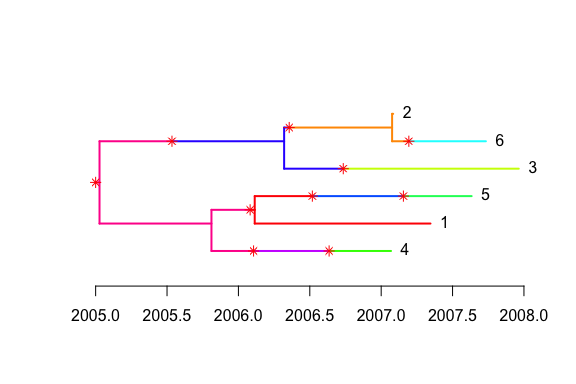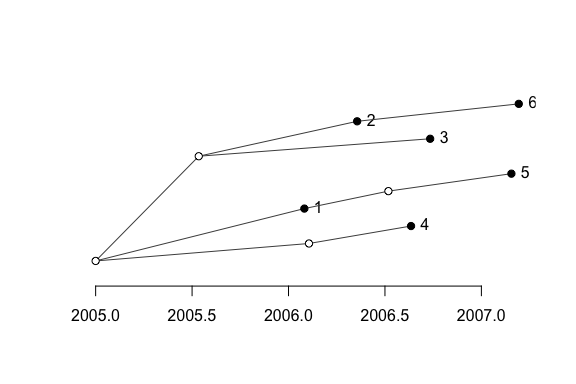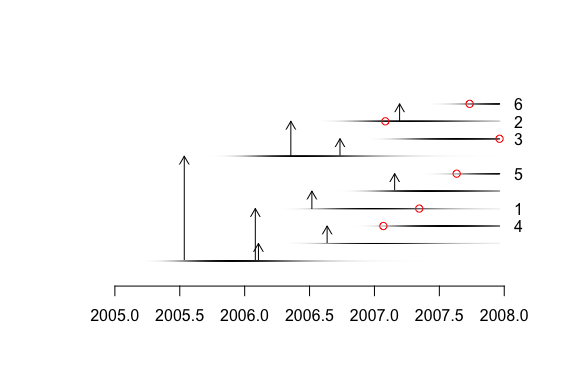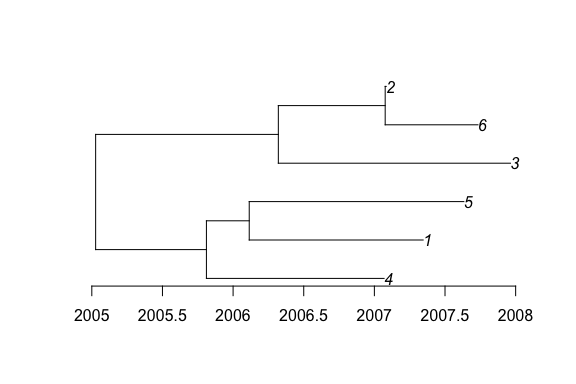# Simulation of outbreak data

#### 2021-04-20

If you want to reproduce exactly the same results as the ones shown in this tutorial, you need to set the seed of your random number generator to zero:

library(TransPhylo)
set.seed(0)

A pathogen has an effective within-host population size of $$N_e=100$$ and a generation time $$g=1$$ day, so that $$N_e g=100/365$$ year. The offspring distribution is negative binomial with mean equal to the basic reproduction number $$R=5$$. Both the generation time and the sampling time are Gamma distributed with parameters (10,0.1) which has a mean of 1 year. The density of sampling is $$\pi=0.25$$. The following commands specify these parameters:

neg=100/365
off.r=5
w.shape=10
w.scale=0.1
pi=0.25

We simulate an outbreak that starts in 2005 and which and is observed up to 2008:

simu <- simulateOutbreak(neg=neg,pi=pi,off.r=off.r,w.shape=w.shape,
w.scale=w.scale,dateStartOutbreak=2005,dateT=2008)

This simulation contains both the transmission tree between infected hosts and the within-host phylogenetic tree of each host. This can be visualised as a colored phlogenetic tree, where each host is represented by a unique color:

plot(simu)The transmission tree can be extracted and plotted separately from the phylogeny:

ttree<-extractTTree(simu)
plot(ttree)A more detailed plot can be displayed as follows:

plot(ttree,type='detailed',w.shape,w.scale)The phylogenetic tree can be extracted and plotted separately from the transmission tree:

ptree<-extractPTree(simu)
plot(ptree)The extracted phylogenetic tree can also be converted into a phylo object from the ape package:

library(ape)
p<-phyloFromPTree(ptree)
plot(p)
axisPhylo(backward = F)You can save this tree into a Newick file for further analysis. This is the tree that is used as the starting poit of the tutorial on inference of a transmission tree from a dated phylogeny.

write.tree(p,'tree.nwk')

The content of this Newick file is:

write.tree(p,'')
##  "((4:1.257652937,(1:1.231048819,5:1.519248672):0.303038892):0.784065883,(3:1.643413444,(6:0.656820028,2:0.007344035611):0.7562780805):1.293120815);"

This phylogeny is scaled in years, but time is measured only relatively to the date of the last sample which is at 0 on the x-axis of the figure above. To use this tree again we also need to know exactly when was the last sample taken:

dateLastSample(simu)
##  2007.964